# Optimal Design and Performance Comparison of a Combined ANFIS-PID with Back Stepping Technique, Using Various Meta-Heuristic Algorithms to Solve Wheeled Mobile Robot Trajectory Tracking Problem

Optimal Design and Performance Comparison of a Combined ANFIS-PID with Back Stepping Technique, Using Various Meta-Heuristic Algorithms to Solve Wheeled Mobile Robot Trajectory Tracking Problem

Rafik Euldji Noureddine Batel Redha Rebhi Giulio Lorenzini Nantapat JarasthitikulchaiYounes Menni Hijaz Ahmad Houari Ameur Weerawat Sudsutad

Laboratory of Advanced Electronic Systems (LSEA), Department of Electrical Engineering, Faculty of Technology, University Yahia Fares, Medea 26000, Algeria

Department of Mechanical Engineering, Faculty of Technology, University of Medea, Medea 26000, Algeria

Department of Engineering and Architecture, University of Parma, Parco Area delle Scienze, 181/A, 43124 Parma, Italy

Department of General Education, Faculty of Science and Health Technology, Navamindradhiraj University, Bangkok 10300 Thailand

Department of Technology, University Centre of Naama (Ctr Univ Naama), P.O. Box 66, Naama 45000, Algeria

Section of Mathematics, International Telematic University Uninettuno, Corso Vittorio Emanuele II, 39, Roma 00186, Italy

Department of Statistics, Faculty of Science, Ramkhamhaeng University, Bangkok 10240, Thailand

Corresponding Author Email:
nantapat.j@nmu.ac.th
Page:
281-298
|
DOI:
https://doi.org/10.18280/jesa.550301
15 January 2022
|
Accepted:
24 May 2022
|
Published:
30 June 2022
| Citation

OPEN ACCESS

Abstract:

The present investigation suggests a novel control technique that merge the advantage of the adaptive Neuro- Fuzzy inference system (ANFIS) with the proportional integral derivative (PID) controller, abbreviated as (ANFIS-PID), for dealing with the dynamics of the of wheeled mobile robot (WMR). A comparative study of various meta-heuristic optimization algorithms is made. The results revealed the highest efficiency of the suggested ANFIS-PID technique compared to seven designed PID controllers, in terms of settling and rise time, overshoot, and the integral square error (ISE) as a cost function. Various cases, study (circular path, diamond path, zigzag path) have highlighted the over performance of mentioned controller. Moreover, this hybrid technique is fused with back-stepping approach for the kinematic control. A lemniscate and a square trajectory were performed to clarify the capability of the mentioned controller.

Keywords:

wheeled mobile robot (WMR), trajectory tracking, back-stepping, proportional integral derivative controller (PID), adaptive neuro-fuzzy inference system (ANFIS), meta-heuristics

1. Introduction

Nowadays, the tracking control issue in the robotics field still challenging and fascinates many researchers. Specifically for the wheeled mobile robot (WMR), since it covers a large civilian and military demands such as agriculture, manufacturing, services, medical, rescue and combat [1-5].

Each application has a special constraint connected to the robot composition (non-holonomic properties of WMRs), Moreover, WMRs are disturbed by task conditions, external load interruption, wheel slips, feedback sensors, which make the design of a precise control technique more difficult . For that purpose, the investigating for new highly precise regulation schemes is required.

In order to overcome this issue, new control methods have been suggested in the last decade. These techniques can be classified into two parts and described by the kinematic or dynamic models . The first group of researchers introduces a basic PID controller to handle the dynamics problem of the robot mobile and concentrating on developing the kinematic controller, an adaptive technique based on neural network using gradient decent for the auto-tuning the parameters for the back-stepping controller is demonstrated in Ref. , fuzzy logic controller for the self-tuning of the kinematic controller in Ref. , and a radial basis function network has been utilized in Ref. .

The major reason behind preferring this kind of controllers is that the dynamic controller’s design is more complex compared to the kinematic one, while the dynamics depend on robot internal components such as the moment of inertia, mass, frictions . However, the underestimating of the complete dynamics knowledge produce insufficient control technique, a torque controller is essential in many applications, like high-speed motions and large transportation . For that reason, a sliding mode controller is introduced in references [12-14], an online real parameters identification of the WMR dynamics is suggested by Martins et al. , an adaptive back-stepping controller in reference , using an adaptive neural network controller for treating unmodeled dynamics in references [17, 18], fuzzy logic controller has been applied in reference [19, 20].

The PID control technique is one of the highly practical and most generally adopted approaches in various industrial applications because of the simplicity structure and good performance . However, optimizing the parameter of the controller is challenging, especially for MIMO systems applications, to overcome this issue, many studies prove the high capability of meta-heuristic algorithms in dealing with this problem. We distingue, Genetic Algorithms (GA) , using particle swarm optimizer (PSO) , whale optimizer (WOA) , grey wolf optimizer (GWO) , using Henry gas solubility optimizer in reference , artificial bee colonies (ABC) .

Generally, the fixed parameters reduce the control performance . In order to overcome this problem, many researchers focusing on the inventing of new modified PID, like integrating the neural network with traditional PID controller (NN-PID) in references [29-31]. This concept gets the simplicity of a PID controllers and the capacity of understanding, adaptability and treating with nonlinearity from neural networks. Other researchers aiming to use the fuzzy logic controller as a tuning method for adjusting PID gains [32-34], a Reinforcement Learning method, have been utilized in reference . However, the need for new regulation mechanisms growing higher and higher in accordance to complexity of the working environment.

The ANFIS could solve this problem by combining fuzzy logic controller advantage to deal with the uncertainties with the ability of neural networks to learn from plants/processes, this concept has attracted an exceptional attention in various engineering fields [36-40]. In this context, several hybrid designs have been made to enhance the robustness of the ANFIS controlled system, by benefiting from advantage and simplicity of a PID controller, we distingue the following mergers: hybrid ANFIS-PID [41-43], (ANFIS+I) controller optimizing using multidimensional PSO for Quadrotor Position Control , (ANFIS-PD+I) controller for robot manipulator .

The purpose of this work is to design a new hybrid optimized controller (ANFIS with PID) by using the grey wolf optimizer (GWO). The suggested controller is abbreviated as GWO-ANFIS-PID controller and it combines the advantages of the PID controller with the ANFIS controller to deal with the speed and angle control of the robot mobile. Moreover, a back-stepping kinematic controller is located in external loop for the regulation of the X, Y and theta coordinates. The following points present the main contributions of our work:

Introducing seven new meta-heuristics techniques to optimize the PID controller, based on the objective function under the name integral square error (ISE). Where these techniques are: particle swarm optimizer (PSO), grey wolf optimizer algorithm (GWO), dragonfly algorithm (DA), ant lion optimizer (ALO), grasshopper algorithm (GOA), moth flame optimizer (MFO), and the artificial bee colony algorithm (ABC).

Several tests in time domain have been made (such as rise time (tr), settling time (ts), overshoot (Mp), peak, and peak time (tp)) to give judgments between the selected nature inspired algorithms.

Results in MATLAB Shows the best designed PID candidate to realize the new hybrid controller (ANFIS-PID).

Improving the ANFIS-PID gains by the power full algorithm namely, grey wolf optimizer (GWO). Where several cases study (circular path, diamond path and zigzag path) gives the demonstration of the high robustness for the mentioned controller over the seven designed PID controllers.

Combining the ANFIS-PID controller with a Back-stepping approached in order to guarantee a minimum tracking error.

To prove the need for a kinematic controller, two types of paths are selected, the lemniscates and the squares trajectory to highlight the superiority of the designed technique in handling both smooth and sharp shaped trajectory.

The rest of the paper is presented as follows: The complete mathematical model of the wheeled mobile robot is presented in Section 2. The global control strategy is described in Section 3, where the simulation findings and analyses are summarized in Sections 4 and section 5, the conclusion of the work.

2. Modeling the Wheeled Mobile Robot

The WMR in Figure 1 has two pairs of DC motors, where R represents the driving wheels radius, φL and φR are the left and right spinning angles of wheels, respectively. Separated by a distance L, θ is the robot angle. At the distance d from the mind-point A, where (Xa, Ya) is the coordinate of A in the inertial frame (X,Y), and the coordinates of any position in the robot frame are illustrated by (Xr, Yr).

## 1.png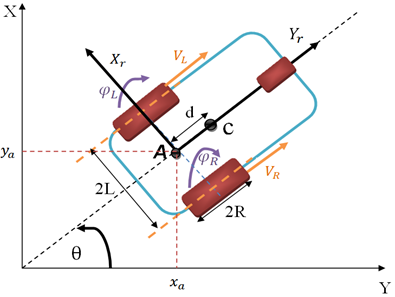Figure 1. Description of the WMR

There are three basic steps to arrive at the complete mathematical model of the WMR: the kinematic model, dynamic model, and finally the DC actuator modeling, which are expressed as follows:

2.1 Kinematic model

This section concentrates on revealing the relationship between the linear and angular velocities of the mechanical processes without analyzing the forces disturbing the motion . The linear speed of each driving wheel is represented as follows:

$\left\{\begin{array}{l}v_{R}=R \dot{\varphi}_{R} \\ v_{L}=R \dot{\varphi}_{R}\end{array}\right.$     (1)

The linear and angular speeds for the WMR are formulated by Eqns. (2) and (3):

$v=\frac{v_{R}+v_{L}}{2}=R \frac{\left(\dot{\varphi}_{R}+\dot{\varphi}_{L}\right)}{2}$     (2)

$\omega=\frac{v_{R}-v_{L}}{2 L}=R \frac{\left(\dot{\varphi}_{R}-\dot{\varphi}_{L}\right)}{2 L}$     (3)

The kinematic constraint can express by the following equations :

No slip constraint:

$-\dot{x}_{a} \sin \theta+\dot{y}_{a} \cos \theta=0$     (4)

Pur rolling constraint:

$\dot{x}_{a} \cos \theta+\dot{y}_{a} \sin \theta+L \dot{\theta}=R \dot{\varphi}_{R}$

$\dot{x}_{a} \cos \theta+\dot{y}_{a} \sin \theta-L \dot{\theta}=R \dot{\varphi}_{L}$     (5)

The three constraint equations are:

$\Lambda(q) \dot{q}=0$      (6)

where:

$(q)=\left[\begin{array}{ccccc}-\sin \theta & \cos \theta & 0 & 0 & 0 \\ \cos \theta & \sin \theta & L & -R & 0 \\ \cos \theta & \sin \theta & -L & 0 & -R\end{array}\right]$     (7)

and

$\dot{q}=\left[\dot{x}_{a} \dot{y}_{a} \dot{\theta} \dot{\varphi}_{R} \dot{\varphi}_{L}\right]^{T}$     (8)

So, the kinematic model obtained is:

$\left[\begin{array}{c}\dot{x}_{a} \\ \dot{y}_{a} \\ \dot{\theta} \\ \dot{\varphi}_{R} \\ \dot{\varphi}_{L}\end{array}\right]=\left[\begin{array}{cc}\cos \theta & 0 \\ \sin \theta & 0 \\ 0 & 1 \\ \frac{1}{R} & \frac{-L}{R} \\ \frac{1}{R} & \frac{-L}{R}\end{array}\right]\left[\begin{array}{l}v \\ \omega\end{array}\right]=\frac{1}{2}\left[\begin{array}{cc}\cos \theta & 0 \\ \sin \theta & 0 \\ 0 & 1 \\ \frac{1}{R} & \frac{-L}{R} \\ \frac{1}{R} & \frac{-L}{R}\end{array}\right]\left[\begin{array}{c}\dot{\varphi}_{R} \\ \dot{\varphi}_{L}\end{array}\right]$      (9)

This may be written as:

$\dot{q}=S(q)_{\eta}$      (10)

where, $\eta=\left[\begin{array}{l}\dot{\varphi}_{R} \\ \dot{\varphi}_{L}\end{array}\right]$  is the vector of the angular velocities of two wheels.

2.2 Dynamical model

The dynamic model purpose describes the study of the relationship between many forces and energies influencing on the process. The interpretation of the robot mechanism is expressed in terms of its component parts, such us the inertia, and centre of mass. Where The Lagrangian equation is represented by Ben Jabeur et al. [34, 46]:

$M(q) \ddot{q}+V(q, \dot{q}) \dot{q}+F(\dot{q})+G(q)+\tau_{d}$$=B(q) \tau-\Lambda^{T}(q)^{\lambda} (11) For simulation purposes and control, Eq. (11) should be transformed into an alternative form, using the kinematic model (10): S^{T}(q) \Lambda^{T}(q)=0 (12) The new matrices are illustrated as following: \left\{\begin{array}{c}\bar{M}(q)=S^{T}(q) M(q) S(q) \\ \bar{V}=S^{T}(q) M(q) \dot{S}(q)+S^{T}(q) V(q, \dot{q}) S(q) \\ \bar{B}=S^{T}(q) B(q)\end{array}\right. (13) The simplified structure of the dynamic equations is given as: \{\bar{M}(q) \dot{\eta}+\bar{V}(q, \dot{q}) \eta=\bar{B}(q) \tau (14) where, \bar{M}(q)=\left[\begin{array}{cc}I_{w}+\frac{R^{2}}{4 L^{2}}\left(m L^{2}+I\right) & \frac{R^{2}}{4 L^{2}}\left(m L^{2}-I\right) \\ \frac{R^{2}}{4 L^{2}}\left(m L^{2}-I\right) & I_{w}+\frac{R^{2}}{4 L^{2}}\left(m L^{2}+I\right)\end{array}\right] (14a) and \bar{V}(q, \dot{q})=\left[\begin{array}{cc}0 & \frac{R^{2}}{2 L} m_{c} d \dot{\theta} \\ -\frac{R^{2}}{2 L} m_{c} d \dot{\theta} & 0\end{array}\right], \bar{B}(q)=$$\left[\begin{array}{ll}1 & 0 \\ 0 & 1\end{array}\right]$       (14b)

Eq. (14) may be rewritten in a compact form:

$\left\{\begin{array}{c}\left(m+\frac{2 I_{w}}{R^{2}}\right) \dot{v}-m_{c} d \omega^{2}=\frac{1}{R}\left(\tau_{R}+\tau_{L}\right) \\ \left(I+\frac{2 L^{2}}{R^{2}} I_{w}\right) \omega+m_{c} d \omega v=\frac{L}{R}\left(\tau_{R}-\tau_{L}\right)\end{array}\right.$        (15)

where, $m=m_{c}+2 m_{w}$ is the total mass of the robot.

$I=I_{c}+m_{c} d^{2}+2 m_{w} L^{2}+2 I_{m}$ is the total equivalent inertia.

2.3 Actuator modeling

The job of the electrical actuator is to drive the mechanical system of a robot. In this work, two pairs of DC motor are used in order to guide the wheels there a determined motion (Figure 2).

## 2.pngFigure 2. Equivalent electrical scheme of the motor.

The dynamic model of the actuators can be represented as :

$\left\{\begin{array}{c}v_{a}=R_{a} i_{a}(t)+L_{a} \frac{d i_{a}(t)}{d t}+e_{a}(t) \\ e_{a}(t)=K_{b} \omega_{m}(t) \\ \tau_{m}=j \frac{d w_{m}(t)}{d t}+f w_{m}(t)+K_{t} i_{a}(t) \\ \tau=N \tau_{m}\end{array}\right.$      (16)

where, ia is the armature current, (Ra, La) is the resistance and inductance of the armature winding, respectively, ea is the back emf, ωm is the rotor angular speed, τm is the motor torque, (Kt, Kb) are the torque constant and back emf constant, respectively, N is the gear ratio, and τ is the output torque applied to the wheel Since the robot motors are mechanically coupled to wheels through the gears. Therefore, each dc motor will have:

For the two motors, the dynamic model is expressed as:

\left\{\begin{aligned} \omega_{m R}=N \dot{\varphi}_{w R} & \text { and } \tau_{R}=N \tau_{m R} \\ \omega_{m L}=N \dot{\varphi}_{w L} & \text { and } \tau_{L}=N \tau_{m L} \end{aligned}\right.      (17)

$\left\{\begin{array}{l}\frac{1}{\left(R+L_{a} P\right)}\left(e_{a r}-K_{b} N \dot{\varphi}_{w R}\right)=i_{R} \text { with } \tau_{R}=N k_{t} i_{R} \\ \frac{1}{\left(R+L_{a} P\right)}\left(e_{a l}-K_{b} N \dot{\varphi}_{w L}\right)=i_{L} \quad \text { with } \tau_{L}=N k_{t} i_{L}\end{array}\right.$       (18)

The Physical parameters of the robot are presented in Ref. .

3. Controller Design and Strategy

Figure 3 explains the simplified form of the general control concept based of the WMR dynamics and kinematics. The role of path generator is to supplies the desired variable x, y and θ, a back-stepping act as a kinematic controller, located in the external loop, this controller measures the difference between the recommended signals (taken from the bloc trajectory generator) and real value from the robot, at next realizes its own linear speed and angle which they are passed to the internal loop, where two ANFIS-PID controller deal with the dynamics. Here, the two dynamic controllers give their own angular and linear speeds based on the data selected from the kinematic controller.

## 3.png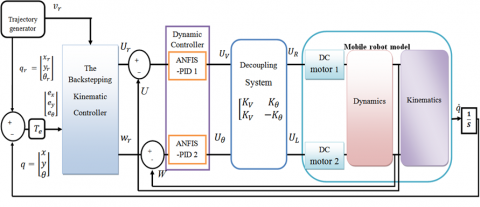Figure 3. General control scheme of the WMR

3.1 Kinematic controller design

In order to reach at a nearly zero distance error, a kinematic controller is required. The major advantage of the back-stepping approach is its practical and simplicity structure, since it will depend only on the kinematic model. Moreover, it has been classified as a stable tracking control law, where other techniques, such as nonlinear feedbacks and sliding-mode techniques, demand the knowledge of the dynamic model and their hardware realization is difficult to implement. For that reason, this controller makes it adopted in our study . The controller structure is introduced in Figure 3, where the input error and velocity vector (vc) are:

$\left[\begin{array}{l}e_{x} \\ e_{y} \\ e_{\theta}\end{array}\right]=\left[\begin{array}{ccc}\cos \theta & \sin \theta & 0 \\ -\sin \theta & \cos \theta & 0 \\ 0 & 0 & 1\end{array}\right]\left[\begin{array}{cc}X_{r}- & X \\ Y_{r}- & Y \\ \theta_{r}- & \theta\end{array}\right]=T_{e} e_{r}$       (19)

$v_{c}=\left[\begin{array}{c}v_{r} \cos \left(e_{\theta}\right)+k_{x} e_{x} \\ w_{r}+k_{y} v_{r} e_{y}+k_{\theta} v_{r} \sin \left(e_{\theta}\right)\end{array}\right]$       (20)

where, kx, ky and kθ are tuning parameters.

3.2 The PID dynamic controller

The PID is a feedback controller that is widely utilized in various applications, especially for robotics and automation industry due to its simplicity structure and reliability that usually leads to satisfying results [21, 47] (Figure 4).

## 4.png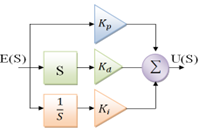Figure 4. Block diagram of the PID controller

The representation of the transfer function for the PID in the time domain (Eq. (21)) and the transfer function in the Laplace domain (Eq. (22)).

$u(t)=K_{p} e(t)+k_{i} \int_{0}^{t} e(t)+K_{d} \frac{d e(t)}{d t}$      (21)

$C(s)=\frac{U(s)}{E(s)}=K_{p}+\frac{K_{i}}{S}+K_{d} S$       (22)

where, Kp, Ki and Kd are proportional, integral and derivative gains constants, respectively. The proportional constant kp role is to regulate the rise time value. where the purpose of the integral action ki is to eliminate steady-state error, the derivative gain kd for reducing the value of overflow and improving a transient response.

The success in designing PID controller rests to the optimal selection of parameters. The Ziegler–Nichols (Z-N) was supported in adjusting the tuning details for the PID. Today, the tuning methodology reaches a new era for soft computing approaches like the meta-heuristics algorithms. For that reason, the next section will focus on describing the tuning strategies.

3.3 Tuning methodology for PID

The block diagram for WMR tuning strategy is highlighted in Figure 5. While the first PID controller obtains the contrast between the desired and real speed from the right DC motor wheel as an input, where the reference velocity is fixed at: Ur =1 m/s.

## 5.pngFigure 5. Bloc diagram for tuning methodology

The second PID controller in the left wheel examines the difference between the physical and desired directions angle as input. Here, the reference angle is a constant value: θr=0.785 rad.

The following Eq. (23) represent the relation between the input voltage of the right and left DC actuators, respectively UR, UL with the outputs of the first and second controller UV, Uθ, respectively.

$\left\{\begin{array}{l}U_{R}=\frac{U_{V}+U_{\theta}}{2} \\ U_{L}=\frac{U_{V}-U_{\theta}}{2}\end{array}\right.$       (23)

The cost function used in the study is the integral square error (ISE) which is demonstrated by Eq. (24):

$I S E=\left(\int_{0}^{\infty}\left[e_{v}(t)\right]^{2} d t+\int_{0}^{\infty}\left[e_{\theta}(t)\right]^{2} d t\right)$      (24)

where:

$\left\{\begin{array}{l}e_{v}=U_{r}-U_{m} \\ e_{\theta}=\theta_{r}-\theta_{m}\end{array}\right.$     (25)

Ur is the desired velocity, Um the actual velocity, ev the velocity error, θr the desired orientation, θm the measured orientation, and eθ is the orientation error, The optimization algorithms used to tune the parameters (Kp, KI, Kd) are described in the next section.

4. Meta-Heuristic Algorithms

A comparative study has been made to demonstrate the capability of the grey wolf optimizer (GWO), compared to particle swarm optimizer (PSO), dragonfly optimizer (DA), Ant Lion optimizer (ALO), grasshopper algorithm (GOA), moth flame optimizer (MFO), and the artificial bee colony algorithm (ABC). Therefore, a brief description of all algorithms is given:

4.1 Particle Swarm Optimizer (PSO)

Each particle in the swarm updates their positions according to the following equations [48, 49]:

\begin{aligned} V_{i}^{k+1}=w V_{i}^{k}+& C_{1} R_{1}\left(p_{i}^{k}+x_{i}^{k}\right) \\ &+C_{2} R_{2}\left(g b e s t_{i}+x_{i}^{k}\right) \end{aligned}     (26)

$X_{i}^{k+1}=X_{i}^{k}+V_{i}^{k+1}$     (27)

Here, i refer to the particle in the swarm. k the iteration step carried out, w the inertia weight parameter and R1 and R2 are random numbers in the range [0, 1]. The coefficients C1 and C2 are the optimization parameters, X: position vector, and $p_{i}^{k}$: best position information achieved by the ith particle, $g b e s t_{i}$: best position information available in the swarm.

4.2 Dragonfly Algorithm (DA)

This approached was first introduced by Mirjalili , and was inspirited by the dynamic and static behaviour of dragonflies in nature explained in the references [50-52]. The life cycle of dragonflies is divided into two phases: the nymph phase and the adult phase . The natural behaviour of each agent in the swarm obligates the attraction to nurturing sources and distract outward enemies [50, 52].

As demonstrated by Reynolds , the behaviour of swarm follows the following principles [50, 52, 53]:

4.2.1 Separation

This step aims to avoid individuals’ collision with their neighbours that is near to its position, the static swarm defined by the following equation:

$S_{i}=\sum_{j=1}^{n} X-X_{j}$      (28)

Here X and $X_{j}$are the positions of the current individual and the jth neighboring individual, respectively. n is the number of neighboring individuals.

4.2.2 Alignment

The velocity matching between dragonflies of the same group is given by:

$A_{i}=\frac{\sum_{j=1}^{n} V_{j}}{n}-X$      (29)

where, vj is the velocity of neighbouring individual j.

4.2.3 Cohesion

The tendency of members towards the centre of the swarm’s group is known as the cohesion and it is defined as:

$C_{i}=\frac{\sum_{j=1}^{n} X_{j}}{n}-X$      (30)

4.2.4 Attraction to food

In order to survive, all individuals must move toward the food. As presented by this mathematical formula:

$F_{i}=X^{+}-X$      (31)

where, $X^{+}$ shows the position of the food source.

4.2.5 Distraction from enemy

This equation represents the movement of dragonflies far away from the enemy’s sources to survive:

$E_{i}=X^{-}-X$      (32)

where, $X^{-}$ shows the position of the enemy. The position update of each dragonfly for testing another weight solution and receiving another fitness value is gotten by calculating ΔX and X using Eq. (36) and Eq. (37) [50, 52]:

$\Delta X_{i}=\left(s S_{i}+a A_{i}+c C_{i}+f F_{i}+e E_{i}\right)+w$       (33)

$X_{t+1}=X_{t}+\Delta X_{t+1}$      (34)

$e=0.1-1 *\left(\frac{0.1}{\frac{I}{2}}\right)$      (35)

$w=0.9-i *\left(\frac{0.9-0.4}{I}\right)$      (36)

where, s, a, c, f, e, and w are the weights of their correspondent element. w is calculated using Eq. (36) here i is the current iteration and I is the number of iterations, and e is calculated in Eq. (35). s, a, and c are three different random numbers between 0 and 2e, f is a random number between 0 and 2. More details can be found elsewhere .

4.3 Ant Lion Optimizer (ALO)

This method proposed by Mirjalili  is inspired by the hunting mechanism of antlions in nature. The antlions have about three years average lifespan that it is spent as larvae except for 3-5 weeks of that period is spent in adulthood . The mechanism is explained elsewhere [55, 56]. The mathematical illustration is given as follows [54-56]:

• Random walks of Ants

X(t)=\left[0, \operatorname{cumsum}\left(2 r\left(t_{1}-1\right), \operatorname{cumsum}\left(2 r\left(t_{2}\right)\right.\right.\right.-1, \cdots, cumsum \left.\left(2 r\left(t_{n}\right)-1\right)\right] (37) where, n is the maximum number of iterations, cumsum calculates the cumulative sum, and t is the step of the random walks. Thus, r(t)= \begin{cases}1 & \text { if rand }>0.5 \\ 0 & \text { if rand }<0.5\end{cases} (38) Here (t) is a stochastic function and rand is a random number generated with uniform distribution at interval of [0, 1]. The positions of ants is saved and utilised during optimisation in the matrix: M_{a n t}=\left[\begin{array}{ccccc}A_{1,1} & A_{1,2} & \cdots & \cdots & A_{1, d} \\ A_{2,1} & A_{2,2} & \cdots & \cdots & A_{2, d} \\ \vdots & \vdots & \vdots & \vdots & \vdots \\ \vdots & \vdots & \vdots & \vdots & \vdots \\ A_{n, 1} & A_{n, 2} & \cdots & \cdots & A_{n, d}\end{array}\right] (39) where, M_{\text {Ant }} is the matrix for saving the position of each ant, A_{i}, shows the value of the j_{w}^{\text {th }} variable of i_{w}^{\text {th }} ant, nis the number of ants, and dis the number of variables. Based on the random walk, each ant updates their position with each step of optimisation. The min-max normalisation equation is used to normalise the random walks: X_{i}^{t}=\frac{\left(X_{i}^{t}-a_{i}\right) \times\left(d_{i}-c_{i}^{t}\right)}{\left(d_{i}^{t}-a_{i}\right)}+c_{i} (40) Here a i is the minimum of random walk of i_{m}^{\text {th }} variable, d_{i} is the maximum of random walk of i_{m}^{\text {th }} variable, c_{i}^{t} is the minimum of i_{\text {th }} variable at \tau_{\text {th }} iteration, and d_{i}^{t} is the maximum of i_{\mathrm{w}}^{\text {th }} variable at \tau_{\mathrm{m}}^{\text {th }} iteration. • Trapping in Antlion’s pit The random walks of ants are influenced by the Antlion’s trap. It is defined as: \begin{aligned} c_{i}^{t} &=\text { Antlion }_{j}^{t}+c^{t} \\ d_{i}^{t} &=\text { Antlion }_{j}^{t}+d^{t} \end{aligned} (41) where, c^{t} represents the minimum of all variables at t^{\text {th }} iteration, d^{t} indicates the vector including the maximum of all variables at t^{\text {h }} iteration, c_{i}^{t} is the minimum of all variables for i^{\text {th }} ant, d_{i}^{t} is the maximum of all variables for i^{\text {th }} ant, and Antlion { }_{j}^{t} shows the position of the selected j^{\text {th }} antlion at t^{\text {th }} iteration. • Building trap During optimisation the algorithm used a roulette wheel operator to choose antlions according to their fitness. This method increased the chance if catching ants. • Sliding ants toward antlion The following equations compute the decrease adaptively to the radius of the random walks hyper sphere of ants: c^{t}=\frac{c^{t}}{I} d^{t}=\frac{d^{t}}{I} (42) where, I is a ratio, c^{t} is the minimum of all variables at t^{\text {th }} iteration, and d^{t} indicates the vector, including the maximum of all variables at t^{\text {th }} iteration. • Catching Pray and Rebuilding the Pit In the final hunting stage, antlions update their position to improve the possibility of catching new prey according to the position of the latest hunted ant: Antlion _{j}^{t}=A n t_{i}^{t} if f\left(A n t_{i}^{t}\right)>f\left(\right. Antlion \left._{j}^{t}\right) (43) Here t represents the current iteration, Antlion { }_{j}^{t} is the position of the selected j^{\text {th }} antlion at t^{\text {th }} iteration, and A n t_{i}^{t} represents the position of i^{\text {th }} ant at t^{\text {th }} iteration. • Elitism Through the whole iteration, the best-obtained antlion is called an elite. This elite influences the movement of the rest of the ants during iteration. So, it is considered that every prey walks randomly around a chosen individual by the roulette wheel and the elite altogether represented by the following equation: A n t_{i}^{t}=\frac{R_{A}^{t}+R_{E}^{t}}{2} (44) where, R_{A}^{t} is the random walk around the antlion selected by the roulette wheel at t^{\text {th }} iteration, R_{E}^{t} is the random walk, and A n t_{i}^{t} represents the position of i^{\text {th }} ant at t^{\text {th }} iteration. 4.4 Artificial Bee Colony (ABC) The artificial bee colony algorithm was first introduced by Karaboga and Basturk  in 2005, while its behaviour  was analysed in 2007. The behaviours of honeybees in finding food sources, splitting the knowledge with the nest, are its main inspiration. The approached classified bees to three types (employed, onlooker, and scout) where each agent plays different roles in the process. More details can be found in reference  while the process of the ABC algorithm is the following : • Initialization At first, a ratio of the population is randomly sprayed into the solution area. Then, the fitness value, the nectar amounts, is fixed. • Move the Onlookers This equation calculates the probability of selecting a food source: P_{i}=\frac{F\left(\theta_{i}\right)}{\sum_{k=1}^{S} F\left(\theta_{k}\right)} (45) where, \theta_{i} denotes the position of the ith employed bee, S represents the number of employed bees, and P_{i} is the probability of selecting the i th employed bee. Later, onlooker bees move by the roulette wheel selection. The amount of nectar is then determined: x_{i j}(t+1)=\theta_{i j}+\phi\left(\theta_{i j}(t)-\theta_{k j}(t)\right) (46) Here x_{i} denotes the position of the ith onlooker bee, t denotes the iteration number, \theta_{k} is the randomly chosen employed bee, j represents the dimension of the solution and \phi(\cdot) produces a series of a random variable in the range [-1, 1]. • Move the Scouts The given equation shows the move of scouts: \theta_{i j}=\theta_{i j \min }+r \cdot\left(\theta_{i j \max }-\theta_{i j \min }\right) (47) where, r is a random number and r \in[0,1]. Next, the best food source obtained so far and the fitness value are updated according to the response, with the respect of termination condition the algorithm outputs the results or repeats the program from step 2 . 4.5 Grey Wolf Optimizer (GWO) The grey wolf algorithm inspired from the social leadership and hunting behaviour of grey wolves in nature [48,49,59]. This approached consist of social hierarchy, enriching prey, search for prey, attacking prey, and hunting. Social hierarchy: The alpha (\alpha) wolf is the leader of wolves, while \operatorname{Beta}(\beta) and delta (\delta) wolves are the second and third levels in the group, respectively. Those three wolves are considered as the best solution to lead the rest wolves known as omega (\omega) wolves toward promising areas in order to find the global solution. d=\left|c \cdot x_{p}(n)-x(n)\right| (48) x(n+1)=x_{p}(n)-a \cdot d (49) where, \mathrm{x}_{\mathrm{p}} : position vector of the prey, \mathrm{n} : current iteration, and \mathrm{x} : position vector of a grey wolf. The coefficient vectors a and c are defined as follows: \vec{a}_{(.)}=2 \vec{l} \cdot \vec{r}_{1}-\vec{l} (50) \vec{c}_{(.)}=2 \cdot \vec{r}_{2} (51) Here, r1 and r2 are random numbers in [0, 1]. The following equations illustrate the mechanism of hunting: \left\{\begin{array}{l}\vec{d}_{\alpha}=\left|\vec{c}_{1} \cdot \vec{x}_{\alpha}-\vec{x}\right| \\ \vec{d}_{\beta}=\left|\vec{c}_{2} \cdot \vec{x}_{\beta}-\vec{x}\right| \\ \vec{d}_{\delta}=\left|\vec{c}_{3} \cdot \vec{x}_{\delta}-\vec{x}\right|\end{array}\right. (52a) \left\{\begin{array}{l}\vec{x}_{1}=\vec{x}_{\alpha}-\vec{a}_{1} \cdot\left(\vec{d}_{\alpha}\right) \\ \vec{x}_{2}=\vec{x}_{\beta}-\vec{a}_{2} \cdot\left(\vec{d}_{\beta}\right) \\ \vec{x}_{3}=\vec{x}_{\delta}-\vec{a}_{3} \cdot\left(\vec{d}_{\delta}\right)\end{array}\right. (52b) x(n+1)=\frac{\vec{x}_{1}+\vec{x}_{2}+\vec{x}_{3}}{3} (52c) 4.6 Grasshoppers Optimization Algorithm (GOA) The Grasshoppers Optimization Algorithm simulated by  is inspired from the natural flocking behaviour of grasshoppers. Those insects are regarded as pests because they damage agricultural corps. The swarming behaviour can be mathematically explained by the following equations [60-62]: X_{i}=S_{i}+G_{i}+A_{i} (53) where, Xi is the position of the ith grasshopper, Si is the classical interaction, Gi is the gravity force on the ith grasshopper, and Ai is the wind advection. The classical interaction is given by: S_{i}=\sum_{j=1}^{N} s\left(d_{i j}\right) \hat{d}_{i j} (54) d_{i j}=\left|x_{i}-x_{j}\right| (55) \hat{d}_{i j}=\frac{x_{j}-x_{i}}{d_{i j}} (56) Here, dij is the distance between the ith and jth grasshoppers, and s is a function for the definition of the strength of social forces defined as follows: s(r)=f e^{-\frac{r}{l}}-e^{-r} (57) where, f is the intensity of attraction, and l is the attractive length scale. The distance between grasshoppers ranges between 0 and 15. Repulsion is observed in the interval [0 2.079]. The grasshoppers enter the comfort zone if they are far from 2.079 units from other grasshoppers. G component is defined as follows: G_{i}=-g \hat{e}_{g} (58) Here, g is the gravitational constant and \hat{e}_{g} is a unity vector towards the center of the earth. Parameter A is given by: A_{i}=u \hat{e}_{w} (59) where, \mathrm{u} is a constant drift and \hat{e}_{w} is a unit vector in the direction of the wind. The position is updated to a new position based on the common position of the grass-hoppers, the food source position, and the position of all other grasshoppers: X_{i}=\sum_{\substack{j=1 \\ j \neq i}}^{N} s\left(\left|x_{j}-x_{i}\right|\right) \frac{x_{j}-x_{i}}{d_{i j}}-g \hat{e}_{g}+u \hat{e}_{w} (60) Here, N is the number of grasshoppers. Although, this Eq. (65) cannot be straight-forward applied for optimization because grasshoppers do not converge to a specified point. Hence, the following is a corrected equation to update the grasshopper’s position: X_{i}^{d}=c\left|\sum_{\substack{j=1 \\ j \neq 1}}^{N} c \frac{u b_{d}-l b_{d}}{2} s\left(\left|x_{j}^{d}-x_{i}^{d}\right|\right) \frac{x_{j}-x_{i}}{d_{i j}}\right|+\widehat{T}_{d} (61) C=\left(C_{\max }-1\right) \frac{C_{\max }-C_{\min }}{M a x I t r} (62) where, u b_{d} is the upper bound, l b_{d} is the lower bound, \widehat{T}_{d} is the value of the \mathrm{D}_{\mathrm{th}} dimension in the target space (optimal solution found so far), and \mathrm{c} is a decreasing coefficient to shrink the comfort zone, repulsion zone, and attraction zone. 4.7 Moth Flame Optimization (MFO) Algorithm The Moth-flame optimization algorithm was first introduced by Mirjalili . Those fancy insets are quite similar to the butterfly family. The natural behaviour of them in navigation known as transverse orientation is the main motivation for the algorithm. Moths fly using a fixed angle with respect to the moon, which is a very efficient mechanism for long travelling distances in a straight line. The MFO algorithm follows the following steps: M F O=(R, V, T) (63) moths randomly and also the fitness values of them, V is a main function that makes the moths move around the search space and T is a termination criterion flag. In V function, the position of each moth with regard to a flame is updated as per Eq. (64): M_{i}=S\left(M_{i}, G_{j}\right) (64) where, S is the spiral function, Mi is the ith moth, and Gj is the jth flame, whereas S\left(M_{i}, G_{j}\right) is calculated using the following equations: S\left(M_{i}, G_{j}\right)=D_{i} e^{b l} \cos (2 \pi l)+G_{j} (65) Here, Di represents distance of ith moth from jth flame, b is constant for announcing the shape of logarithmic spiral, and l is a random number between [− 1, 1]. D is calculated using the following equation. D_{i}=\left|G_{j}-M_{i}\right| (66) where, G_{j} indicate the j_{m}^{\text {th }} flame and M_{i} is the i_{m}^{\text {th }} moth. The position of moths is updated with respect to \mathrm{n} different locations in the search space, which can degrade the best promising solutions exploitation. Consequently, the number of flames adaptively decreases over the course of iterations using the following formula: \operatorname{flame}_{n o}=\operatorname{round}\left(F N-1 * \frac{N-1}{I N}\right) (67) where, I is the current number of iterations, I N is the maximum number of iterations and FN is the maximum number of flames. More details can be found elsewhere [63-65]. 5. The ANFIS Controller Description Adaptive Network-Based Fuzzy Inference System (ANFIS) is a hybrid soft computing algorithm that combined the neuro-fuzzy technique, namely fuzzy inference system (FIS) with artificial neural network (ANN). ANFIS was firstly introduced by Jang  and it is based on the first-order Sugeno fuzzy model .The ANFIS approaches integrate the best feature of fuzzy systems and neural networks by exercising learning ability and capability of FIS and ANN, respectively . The ANFIS architecture consists of if-then rules and couples of input-output data of fuzzy. For training, ANFIS uses neural network learning algorithms. As the algorithm used it to fine-tune the membership function (MF) and the associated parameter that methods the desired data sets [68, 69]. For clarifying, a Sugeno fuzzy model with two inputs, x and y, and one output is considered. Normally, the fuzzy rules are reported as follows [66-69]: Rule I: if x=A1 and B1, then f_{1}=p_{1} x+q_{1} y+r_{1} (68) Rule II: if x=A2 and y=B2, then f_{2}=p_{2} x+q_{2} y+r_{2} (69) where, f is an output parameter (response), p, q, & r are linear parameters, and A&B are nonlinear parameters. Figure 6 Illustrates the five layers used to create ANFIS structure while the function of each layer is represented as follows: ## 6.png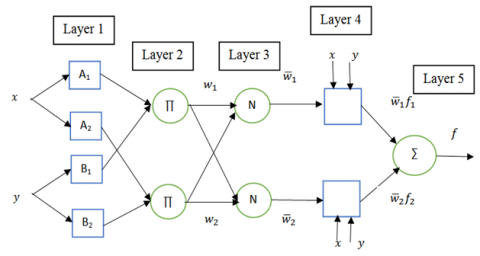Figure 6. The ANFIS architecture with two input parameters x and y Layer 1. Input nodes: the nodes of this layer generate membership grades to which they belong to each of the relevant fuzzy sets using MFs. \left\{\begin{array}{l}O_{i}^{1}=\mu_{A i}(x) \\ O_{i}^{2}=\mu_{B i}(y)\end{array}\right. (70) Here, i= 1, 2, x, y are the crisp inputs to node i, and Ai &Bi. The linguistic label (small, large, etc.) associated with the node function. µAi, µBi respectively. Typically, µAi (or µBi) is selected as: \mu_{A i}(x)=\frac{1}{1+\left[\left(x-c_{i} / a_{i}\right)^{2}\right]^{b_{i}}} (71) or \mu_{A i}(x)=\exp \left\{-\left(\frac{x-c_{i}}{a_{i}}\right)^{2}\right\} (72) where, {ai, bi, ci} are the premise parameter set. Layer 2. Rule nodes: The firing strength of each rule is calculated with mathematical multiplication. For instance, O_{i}^{2}=w_{i}=\mu_{A_{i}}(x) \cdot \mu_{B_{i}}(y) (73) Each node output represents the firing strength of a rule. Layer 3. Average nodes: the ith node calculates the ratio of the ith rule firing strength to the summation of the firing strengths of all rules consequently, defined as: O_{i}^{3}=\bar{w}_{i}=\frac{w_{i}}{w_{1}+w_{2}} (74) wi is taken as the normalized firing strength. Layer 4. Consequent nodes: The node function of the fourth layer calculates the contribution of each ith rules toward the overall output, as given: O_{i}^{4}=\bar{w}_{i} f_{i}=\bar{w}_{i}\left(p_{i} O_{i}^{4}=\bar{w}_{i} f_{i}\right.=\bar{w}_{i}\left(p_{i} x+q_{i} y+r_{i}\right)       (75)

where, $\bar{w}_{i}$ isthe output of the layer 3, and {pi, qi, ri} the parameter set.

Layer 5. Output nodes: The single-node computes the overall output by adding all the incoming signals from the 4th layer:

$O_{i}^{5}=$ overall output $=\sum_{i} \bar{w}_{i} f_{i}=\frac{\sum_{i} w_{i} f_{i}}{\sum_{i} w_{i}}$       (76)

The final output of ANFIS can be given as:

$f_{\text {out }}=\bar{w}_{1} f_{1}+\bar{w}_{2} f_{2}=\frac{w_{1}}{w_{1}+w_{2}} f_{1}+\frac{w_{2}}{w_{1}+w_{2}} f_{2}$$=\left(\bar{w}_{1} x\right) p_{1}+\left(\bar{w}_{1} y\right) q_{1}+\left(\bar{w}_{1}\right) r_{1}$$+\left(\bar{w}_{2} x\right) p_{2}+\left(\bar{w}_{2} y\right) q_{2}+\left(\bar{w}_{2}\right) r_{2}$       (77)

5.1 Designing the ANFIS-PID dynamic controller

The complete control approach that controls the dynamics of the robot mobile based on the ANFIS-PID is divided into three steps:

First step, Figure 7, represent the first step, which indicates the training stage of the ANFIS controller. The ANFIS use their adaptive and learning skills to learn and to predict the best recommended control efforts based on the already data. Which they are obtained from the designed GWO-PID controllers in the previous section.

## 7.pngFigure 7. The ANFIS in training phase

The realized data were saved in a MATLAB/SIMULINK file. From the command anfisedit, these data were taken for training. The complete process was illustrated in Figure 8.

Figure 8 presents the internal composition of the ANFIS controller. The method used for ANFIS training is the hybrid training algorithm, with the input nodes (2, 2, 2), gaussmf membership function for the inputs and linear membership function for the output, each having 8 rules (see Figure 8.b), the epoch length was used is 50 iterations for each sample, with, 0.01s as the Simulink sampling time.

At the end of the training the result was saved as a fis file with respect to Sugeno-style. The root mean square error (RMSE) was found to be 0.1053 and be 0.094977 06 for ANFIS-PID1 and ANFIS-PID2, respectively.

## 8a.png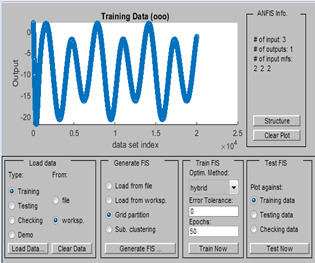(a)

## 8b.png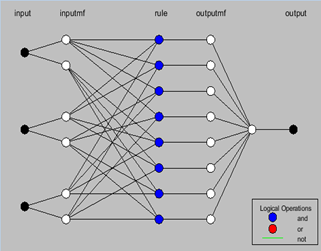(b)

## 8c.png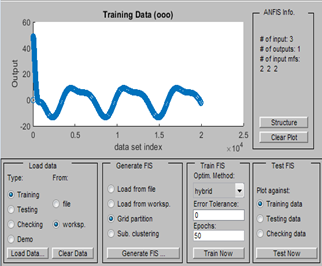(c)

## 8d.png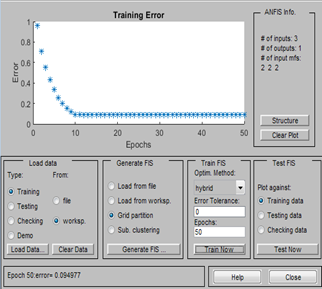(d)

Figure 8. (a) Data configuration for first ANFIS-PID controller, (b) structure of the ANFIS-PID controller, (c) data configuration for second ANFIS-PID controller, (d) error minimization corresponding to the second controller

## 9.png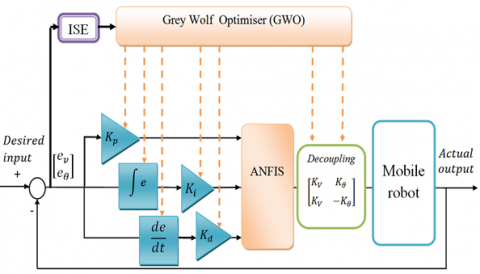Figure 9. Adjusting the parameters for the designed ANFIS-PID controller using GWO

Second step, scaling the new parameters using the GWO optimizer.

After the training step is complete and the optimal data are received, the designed ANFIS-PID controller must be developed by improving the parameters, i.e., Kp, KI, Kd, Kv, Kθ as demonstrated in Figure 9. To find the optimal values of the scaling gains, grey wolf method is proposed to guarantee a minimum ISE value.

Third step, the gained control scheme of the dynamic controller is showed in Figure 10. It reveals the hybridize between the ANFIS and PID controller, abridged as ANFIS-PID. Two trained ANFIS-PID controllers are selected for running the right and left DC motor voltage of the WMR, where a decoupling process is applied to separate the two control actions.

## 10.pngFigure 10. Bloc diagram of the final ANFIS-PID dynamic controller

The first controller takes the difference between the actual and reference speed from the right wheel as an input. The angle controller located in the left wheel studies the difference between the actual and desired orientations as an input.

Eq. (52) describe the relationship between the input voltages of the right and left DC actuators UR and UL, respectively, with the outputs of the first and second controller UV and Uθ, respectively, where the factors of the decoupling system are respectively Kv and Kθ.

$\left\{\begin{array}{l}U_{R}=K_{V} \cdot U_{V}+K_{\theta} \cdot U_{\theta} \\ U_{L}=K_{V} \cdot U_{V}-K_{\theta} \cdot U_{\theta}\end{array}\right.$       (78)

where

$\left\{\begin{array}{l}e_{v}=U_{r}-U_{m} \\ e_{\theta}=\theta_{r}-\theta_{m}\end{array}\right.$       (79)

Ur is the desired velocity, Um the actual velocity, ev the velocity error, θr the desired angle, θm the measured orientation, and eθ is the orientation error.

6. Results and Discussion

This study selected the use of various criteria and evaluations, a comparative study of the convergence curve performance between all controllers, the transition parameters of linear speed and of the angle for all techniques, also several cases study for the path tracking has been created, in order to confirm the over performance and high robustness of the suggested GWO-ANFIS-PID controller, In comparison to GWO-PID, PSO-PID, ALO-PID, GOA-PID, DA-PID, ABC-PID and MFO-PID.

In order to examine the convergence behavior of the nature inspired algorithms, all simulation experiments were executed more than 30 times for each method on a PC separately, the maximum number iterations were 100, and population size is set to 20.

6.1 Convergence curve of all algorithms

Figure 11 highlights the convergence curves of the seven selected optimizations algorithms for designing the PID controller in comparison to our proposed hybrid controller (GWO-ANFIS-PID).

The result proves the over-performance of the GWO-ANFIS-PID controller. The minimum ISE value attained for all approaches used in the study is shown in Table 1. From Figure 11 and Table 1, it’s straightforward that the GWO optimizer gives the lowest ISE value with the faster convergence behavior compared to the other six techniques. Which were made it the best candidate for designing the ANFIS-PID controller. While the proposed GWO-ANFIS-PID controller has the lowest ISE value (0.0889).

## 11.png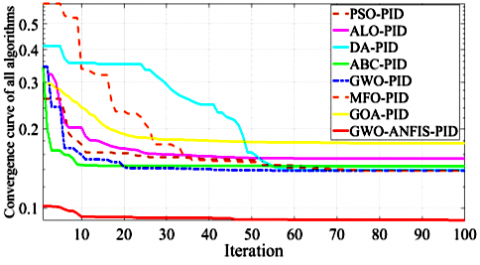Figure 11. Convergence curve of the seven algorithms compared to GWO-ANFIS-PID

Table 1. ISE values of the five algorithms

 algorithms ISE PSO-PID 0.138826 DA-PID 0.139292 ALO-PID 0.154018 ABC-PID 0.143813 GOA-PID 0.176242 MFO-PID 0.138489 GWO-PID 0.138510 GWO-ANFIS-PID 0.089690

6.2 Step response performance of speed controller

Figure 12 reveals the comparative analyses of the step responses performance for the linear speed that was designed with the help of the seven different techniques in comparison to the proposed GWO-ANFIS-PID controller.

## 13.png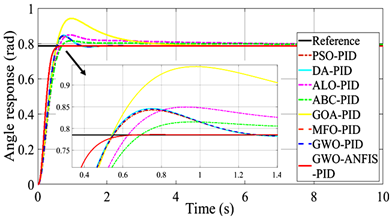Figure 12. Step response comparison for all velocity controllers

Table 2 shows the parameter details of all meta-heuristic approaches, for the linear speed controller, and Table 3 presents the performance achieved in the time domain.

Table 2. The tuned gain details of linear velocity for all controllers

 Methods Speed controller parameter tuning $K_{p}$ $K_{i}$ $K_{d}$ $K_{V}$ $K_{\theta}$ PSO-PID 149.98 97.40 1.013 0.50 0.50 DA-PID 150 1 1 0.50 0.50 ALO-PID 108.46 52.24 0.72 0.50 0.50 ABC-PID 148.78 142.40 1 0.50 0.50 GOA-PID 81.60 89.97 1 0.50 0.50 MFO-PID 149.88 149.97 1 0.50 0.50 GWO-PID 150 148.94 1.001 0.50 0.50 GWO-ANFIS-PID 452.007 13.59 1.25 1.5 0.9

The best overshoot percentage (Mp %) was found by the ABC-PID, and best settling time (ts) is reached by DA-PID. where the GWO-PID controller advantage is the fast rise time and accepted settling time (ts) value obtained, but a high overshoot, this result were shared with the PSO-PID and MFO-PID.

While the GWO-ANFIS-PID controller enclose all advantages of the previous seven techniques by giving the best settling time and an excellent overshoot value and fastest rise time (tr).

6.3 Step response performance of angle controller

Figure 13 describes the comparative analyses of the angle step responses performance that was designed with the aid of the seven previous mentioned approaches in comparison to the recommended GWO-ANFIS-PID controller.

Table 3. Performance characteristic for velocity controller

 Methods Transition parameters for speed control $t_{r}$ Mp% Undershoot Peak $t_{p}$ $t_{s}$ PSO-PID 0.0190 21.3279 3.3018e-05 1.2133 0.2148 1.5344 DA-PID 0.0191 22.2135 3.3017e-05 1.2221 0.2148 0.5248 ALO-PID 0.0221 23.2889 3.5750e-05 1.2329 0.2148 1.9670 ABC-PID 0.0190 17.1998 3.3097e-05 1.1720 0.1933 1.3128 GOA-PID 0.0332 35.0362 7.8275e-05 1.3504 0.2148 2.0684 MFO-PID 0.0189 21.1015 3.3025e-05 1.2110 0.2148 1.4805 GWO-PID 0.0189 21.1147 3.3017e-05 1.2111 0.2148 1.4849 GWO-ANFIS-PID 0.0125 8.8853 0 1.0889 0.1700 0.2547

## 13.pngFigure 13. Comparison of step response for the orientation

From Figure 13, Tables 4 and 5, we can say that the GWO-ANFIS-PID gives a zero-overshoot value, with the best rise time (tr for 10% → 90%) and settling time value (ts for ±2% tolerance), were the GWO-PID comes at the second place. For that reason, our next examinations are concentrating only on analyzing the two best candidates (GWO-PID and GWO-ANFIS-PID).

Table 4. The tuned main parameters of the three controllers for angle control

 Methods angle controller parameter tuning $K_{p}$ $K_{i}$ $K_{d}$ $K_{V}$ $K_{\theta}$ PSO-PID 149.93 1 31.019 0.50 0.50 DA-PID 150 1 30.53 0.50 0.50 ALO-PID 131.90 22.60 33.21 0.50 0.50 ABC-PID 145.24 11.46 37.86 0.50 0.50 GOA-PID 149.95 112.96 41.14 0.50 0.50 MFO-PID 150 1 30.85 0.50 0.50 GWO-PID 150 1.388 30.83 0.50 0.50 GWO-ANFIS-PID 500 1.01 75.31 1.5 0.9

Table 5. Performance characteristics of all angle controllers

 Methods Transition parameters for angle controller $t_{r}$ Mp% Undershoot Peak $t_{p}$ $t_{s}$ PSO-PID 0.3539 7.2995 0 0.8423 0.7449 1.1016 DA-PID 0.3499 7.7670 0 0.8460 0.7449 1.1006 ALO-PID 0.4034 8.1941 0 0.8493 0.9259 6.0690 ABC-PID 0.4247 3.8441 0 0.8152 0.9539 3.1976 GOA-PID 0.3606 20.0551 0 0.9424 0.9826 3.3034 MFO-PID 0.3522 7.5138 0 0.8440 0.7449 1.1013 GWO-PID 0.3518 7.5961 0 0.8446 0.7449 1.1057 GWO-ANFIS-PID 0.2834 0.1047 0 0.7858 0.7700 0.4302

## 14.png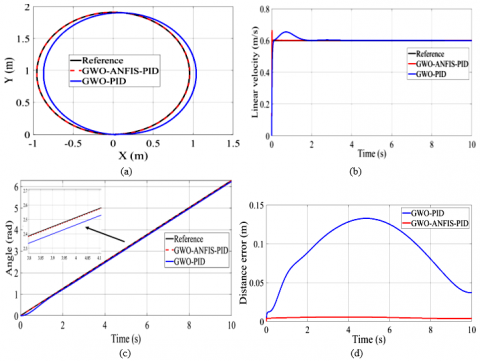Figure 14. (a) Circle trajectory in XY plane, (b) linear velocity response for all controllers, (c) angle response of all controllers, (d) error of the distance

6.4 Circular path

This case, represent a conventional type of smooth trajectory that have been made to confirm the capacity of the GWO-ANFIS-PID controller in dealing with this kind of paths, in comparison to the GWO-PID (Figure 14).

Here, a progressive variation in both linear and angular speed is applied for that purpose, results proves that the GWO-ANFIS-PID controller better handling with smooth trajectory than the GWO-PID controller, due to a minimum tracking error from the suggested controller than the GWO-PID one. Moreover, since the PID controller gives a very high overshoot in the linear velocity response at starting time. Which makes him. unsupported for handling the tracking issue.

6.5 Diamond shape trajectory

The Diamond shaped trajectory is a clear example of a non-continuous gradient path. The main problem of this type of paths is the sharp and a non-continuous motion, which calls for a serious control technique.

The spontaneous changing in velocity and due to the slow rise time and settling time, makes the GWO-PID controller gives slow actions, that causes high distance error and a very high overshoot value too from this controller, that might generate an unstable and a troubled motion, which could by the time damage the DC actuators, for that reason it’s unsuitable for this kind of paths (Figure 15).

The GWO-ANFIS-PID controller has a tolerable overshoot contrasted to the GWO-PID and rapidly time response, and a very small distance error too, which makes it supported for this type of trajectory.

## 15.png(a)

## 15b.png(b)

## 15c.png(c)

## 15d.png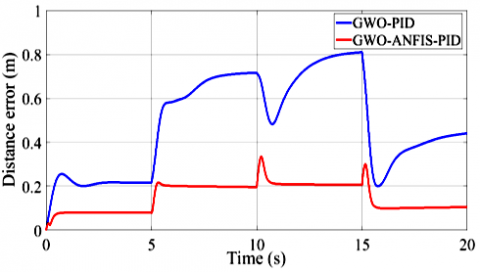(d)

Figure 15. (a) Diamond shape trajectory in XY plane, (b) linear velocity corresponding to controllers, (c) angle response corresponding to the path for the two controllers, (d) error of the distance

6.6 Zig-zag trajectory

## 16a.png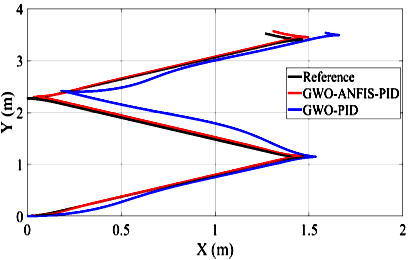(a)

## 16b.png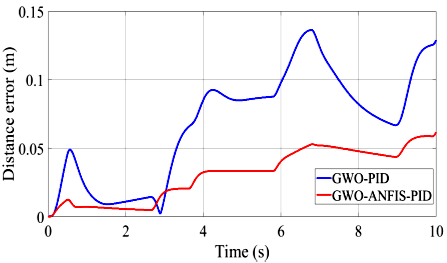(b)

## 16c.png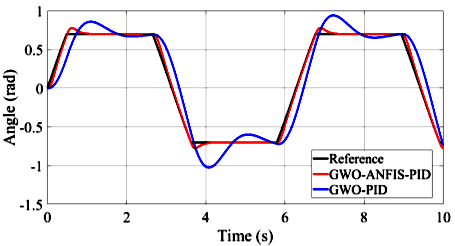(c)

## 16d.png(d)

Figure 16. (a) Zigzag path in XY plane, (b) the tracking error of the controllers, (c) the theta angle response corresponding to the controllers, (d) the linear velocity corresponding to the controllers

Here a trapezoidal signal was applied, for that purpose progressively changing can be seen for both linear speed and angle, the GWO- ANFIS-PID controller gives less overshoot and better time response, contrary to the GWO-PID which gives an important overshoot and a high distance error value (Figure 16).

6.7 Adding the back-stepping controller in the external loop

The designed ANFIS-PID controller role is to guarantee a minimum linear and angular velocity error, which calls for a kinematic controller. Accordingly, a back-stepping controller was suggested to maintain a minimal distance error. The error is less than 0.002 m. To highlight the robustness of the Back-stepping combined with ANFIS-PID controller, a square and a lemniscates trajectory were preferred.

6.7.1 Case of lemniscates

In order to generate this path, the following equation were applied :

$x_{R}(n)=2.5 * \cos \left(2 * \pi * \frac{t}{20}\right)$      (80)

$y_{R}(n)=-2.5 * \sin \left(2 * \pi * \frac{t}{30}\right)$     (81)

$\theta_{R}(n)=\operatorname{atan2}\left[\begin{array}{l}\left(\frac{y_{R}(n)-y_{R}(n-1)}{t+\epsilon}\right) \\ \frac{\left(x_{R}(n)-x_{R}(n-1)\right)}{t+\epsilon}\end{array}\right]$     (82)

where the back-stepping parameters are selected as: kx=10, ky = 80 and kθ = 15.

The desired and physical paths of the lemniscates are presented in Figure 17, displayed in blue and red lines, respectively. Figure 18 (a-d) illustrate the errors of x, y, θ, and the tracking trajectory, respectively. The effect of the included kinematic controller in the external loop is indicated in Figure 18 (d), the distance error is less than 0.002 meter. For that reason, the two trajectories have exactly the same form in Figure 17.

## 17.png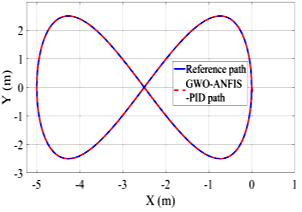Figure 17. Lemniscate path

## 18a.png(a)

## 18b.png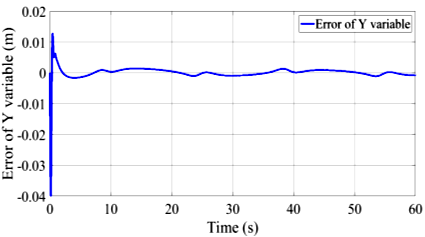(b)

## 18c.png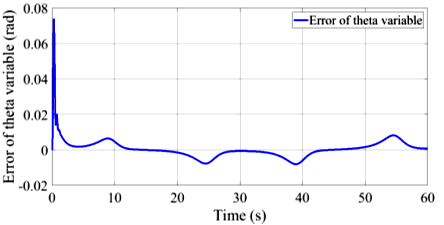(c)

## 18d.png(d)

Figure 18. Error corresponding to (a) x, (b) y, (c) the theta angle, (d) the trajectory tracking

6.7.2 Case of square trajectory

The recommended and actual trajectories of the square path are shown in Figure 19, highlighted in blue and red lines, respectively, Figure 20 (a)-(c) display the errors of x, y, θ, and the tracking distance error, respectively.

## 19.png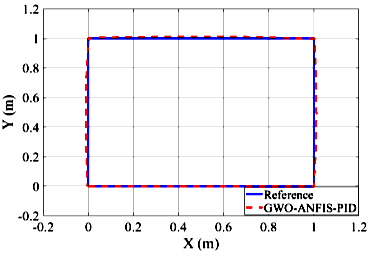Figure 19. Square trajectory

## 20a.png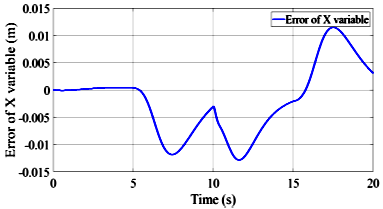(a)

## 20b.png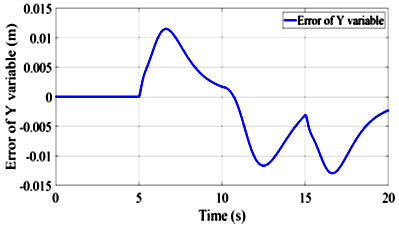(b)

## 20c.png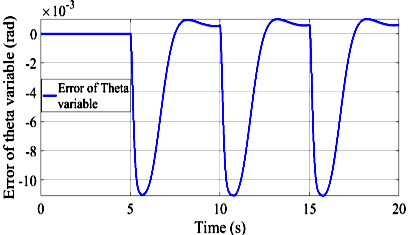(c)

## 20d.png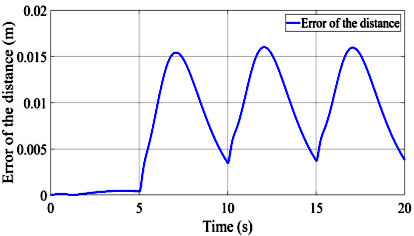(d)

Figure 20. Error corresponding to (a) x, (b) y, (c) the theta angle, (d) the trajectory tracking

The square trajectory is classified as a sharp-shaped path too, therefore it was chosen for this study in order to justify the capability of the proposed hybrid controller, a minimum distance error reached (about 0.015 meter), and an error angle fewer than 0.01 rad, therefore we can see the nearly superposition of the actual on reference path in Figure 19.

7. Conclusions

This paper suggested a new combination technique between the PID controller and an adaptive neuro-fuzzy inference system (ANFIS), shortened to ANFIS-PID controller, to deal with the motion regulation of the wheeled mobile robot (WMR). Tuning parameter of the proposed hybrid controller are challenging tasks, a comparative study of various nature inspired methods are introduced in the study, based on the integral square error (ISE). the selected algorithms namely, particle swarm optimizer (PSO), grey wolf optimizer algorithms (GWO), dragonfly optimizer(DA), ant lion optimizer (ALO), grasshopper algorithm (GOA), moth flame optimizer (MFO), and the artificial bee colony algorithm (ABC), simulation in MATLAB environment gives a demonstration and judgment between the ANFIS-PID controller and these seven techniques ,in terms of lowest overshoot value, minimum ISE value, convergence curve, less peak, peak time, and faster rise time and settling time received. Various study cases have been made, demonstrates the ability and stability of the proposed ANFIS-PID controller tuned by GWO algorithm, which has the capacity to generate smooth and suitable control signals for both linear and angular speeds, great performance over the PID controllers.

Moreover, to guarantee a low tracking error, a back-stepping technique was applied at the kinematic control level, where a lemniscate and a square trajectory have already demonstrated the robustness of the mentioned controller in both smooth and sharp, shaped trajectory.

Acknowledgement

N. Jarasthitikulchai would like to acknowledge financial support by Navamindradhiraj University through the Navamindradhiraj University Research Fund (NURF).

References

 Poberznik, A., Leino, M., Huhtasalo, J., Jyräkoski, T., Valo, P., Lehtinen, T., Kortelainen, J., Merilampi, S., Virkki, J. (2021). Mobile robots and RFID technology-based smart care environment for minimizing risks related to employee turnover during pandemics. Sustain., 13(22): 12809. https://doi.org/10.3390/su132212809

 Saputra, R.P., Rakicevic, N., Kuder, I., Bilsdorfer, J., Gough, A., Dakin, A., de Cocker, E., Rock, S., Harpin, R., Kormushev, P. (2021). Resqbot 2.0: An improved design of a mobile rescue robot with an inflatable neck securing device for safe casualty extraction. Appl. Sci., 11(12): 5414. https://doi.org/10.3390/app11125414

 Catalan, J.M., Blanco, A., Bertomeu-Motos, A., Garcia-Perez, J.V., Almonacid, M., Puerto, R., Garcia-Aracil, N. (2021). A modular mobile robotic platform to assist people with different degrees of disability. Appl. Sci., 11(15): 7130. https://doi.org/10.3390/app11157130

 Kot, T., Novák, P. (2018). Application of virtual reality in teleoperation of the military mobile robotic system TAROS. Int. J. Adv. Robot. Syst., 15(1): 1-6. https://doi.org/10.1177%2F1729881417751545

 Fue, K., Porter, W., Barnes, E., Rains, G. (2020). An extensive review of mobile agricultural robotics for field operations: Focus on cotton harvesting. AgriEngineering, 2(1): 150-174. https://doi.org/10.3390/agriengineering2010010

 Cen, H., Singh, B.K. (2021). Nonholonomic wheeled mobile robot trajectory tracking control based on improved sliding mode variable structure. Wireless Communications and Mobile Computing, 2021: 2974839. https://doi.org/10.1155/2021/2974839

 Abdulwahhab, O.W., Abbas, N.H. (2018). Design and stability analysis of a fractional order state feedback controller for trajectory tracking of a differential drive robot. Int. J. Control. Autom. Syst., 16: 2790-2800. https://doi.org/10.1007/s12555-017-0234-8

 Mohareri, O., Dhaouadi, R., Rad, A.B. (2012). Indirect adaptive tracking control of a nonholonomic mobile robot via neural networks. Neurocomputing, 88: 54-66. https://doi.org/10.1016/j.neucom.2011.06.035

 Khai, T.Q., Ryoo, Y.J., Gill, W.R., Im, D.Y. (2020). Design of kinematic controller based on parameter tuning by fuzzy inference system for trajectory tracking of differential-drive mobile robot. Int. J. Fuzzy Syst., 22: 1972-1978. https://doi.org/10.1007/s40815-020-00842-9

 Khai, T.Q., Ryoo, Y.J. (2019). Design of adaptive kinematic controller using radial basis function neural network for trajectory tracking control of differential-drive mobile robot. Int. J. Fuzzy Log. Intell. Syst., 19(4): 349-359. http://dx.doi.org/10.5391/IJFIS.2019.19.4.349

 Gheisarnejad, M., Khooban, M.H. (2019). Supervised control strategy in trajectory tracking for a wheeled mobile robot. IET Collab. Intell. Manuf., 1(1): 3-9. https://doi.org/10.1049/iet-cim.2018.0007

 Nikranjbar, A., Haidari, M., Atai, A.A. (2018). Adaptive sliding mode tracking control of mobile robot in dynamic environment using artificial potential fields. J. Comput. Robot., 11(1): 1-14.

 Wu, X., Jin, P., Zou, T., Qi, Z., Xiao, H., Lou, P. (2019). Backstepping trajectory tracking based on fuzzy sliding mode control for differential mobile robots. J. Intell. Robot. Syst. Theory Appl., 96: 109-121. https://doi.org/10.1007/s10846-019-00980-9

 Wang, G., Zhou, C., Yu, Y., Liu, X. (2019). Adaptive sliding mode trajectory tracking control for WMR considering skidding and slipping via extended state observer. Energies, 12(17): 3305. https://doi.org/10.3390/en12173305

 Martins, F.N., Celeste, W.C., Carelli, R., Sarcinelli-Filho, M., Bastos-Filho, T.F. (2008). An adaptive dynamic controller for autonomous mobile robot trajectory tracking. Control Eng. Pract., 16(11): 1354-1363. https://doi.org/10.1016/j.conengprac.2008.03.004

 Binh, N.T., Tung, N.A., Nam, D.P., Quang, N.H. (2019). An adaptive backstepping trajectory tracking control of a tractor trailer wheeled mobile robot. Int. J. Control. Autom. Syst., 17: 465-473. https://doi.org/10.1007/s12555-017-0711-0

 Rossomando, F.G., Soria, C., Carelli, R. (2011). Autonomous mobile robots navigation using RBF neural compensator. Control Eng. Pract., 19(3): 215-222. https://doi.org/10.1016/j.conengprac.2010.11.011

 Bozek, P., Karavaev, Y.L., Ardentov, A.A., Yefremov, K.S. (2020). Neural network control of a wheeled mobile robot based on optimal trajectories. Int. J. Adv. Robot. Syst., 17(2): 1-10. https://doi.org/10.1177/1729881420916077

 Das, T., Kar, I.N. (2006). Design and implementation of an adaptive fuzzy logic-based controller for wheeled mobile robots. IEEE Trans. Control Syst. Technol., 14(3): 501-510. https://doi.org/10.1109/TCST.2006.872536

 Štefek, A., Pham, V.T., Krivanek, V., Pham, K.L. (2021). Optimization of fuzzy logic controller used for a differential drive wheeled mobile robot. Appl. Sci., 11(13): 6023. https://doi.org/10.3390/app11136023

 Aldair, A.A., Al-Mayyahi, A., Wang, W. (2020). Design of a stable an intelligent controller for a quadruped robot. J. Electr. Eng. Technol., 15: 817-832. https://doi.org/10.1007/s42835-019-00332-5

 Serradilla, F., Cañas, N., Naranjo, J.E. (2020). Optimization of the energy consumption of electric motors through metaheuristics and PID controllers. Electron., 9(11): 1-16. https://doi.org/10.3390/electronics9111842

 Latha, K., Rajinikanth, V., Surekha, P.M. (2013). PSO-based PID controller design for a class of stable and unstable systems. ISRN Artif. Intell., 2013: 1-11. https://doi.org/10.1155/2013/543607

 Mosaad, A.M., Attia, M.A., Abdelaziz, A.Y. (2019). Whale optimization algorithm to tune PID and PIDA controllers on AVR system. Ain Shams Eng. J., 10(4): 755-767. https://doi.org/10.1016/j.asej.2019.07.004

 Dutta, P., Nayak, S.K. (2021). Grey wolf optimizer based PID controller for speed control of BLDC motor. J. Electr. Eng. Technol., 16(6): 955-961. https://doi.org/10.1007/s42835-021-00660-5

 Ekinci, S., Hekimoğlu, B., Izci, D. (2021). Opposition based Henry gas solubility optimization as a novel algorithm for PID control of DC motor. Eng. Sci. Technol. an Int. J., 24(2): 331-342. https://doi.org/10.1016/j.jestch.2020.08.011

 Bagis, A., Senberber, H. (2017). ABC algorithm based PID controller design for higher order oscillatory systems. Elektron. ir Elektrotechnika, 23(6): 3-9. https://doi.org/10.5755/j01.eie.23.6.19688

 Slama, S., Errachdi, A., Benrejeb, M. (2019). Neural adaptive PID and neural indirect adaptive control switch controller for nonlinear MIMO systems. Mathematical Problems in Engineering, 2019: 7340392. https://doi.org/10.1155/2019/7340392

 Ye, J. (2008). Adaptive control of nonlinear PID-based analog neural networks for a nonholonomic mobile robot. Neurocomputing, 71(7-9): 1561-1565. https://doi.org/10.1016/j.neucom.2007.04.014

 Pei, G., Yu, M., Xu, Y., Ma, C., Lai, H., Chen, F., Lin, H. (2021). An improved PID controller for the compliant constant-force actuator based on bp neural network and smith predictor. Appl. Sci., 11(6): 2685. https://doi.org/10.3390/app11062685

 Xu, S.S.D., Huang, H.C., Chiu, T.C., Lin, S.K. (2019). Biologically-inspired learning and adaptation of self-evolving control for networked mobile robots. Appl. Sci., 9(5): 1034. https://doi.org/10.3390/app9051034

 Mai, T.A., Dang, T.S., Duong, D.T., Le, V.C., Banerjee, S. (2021). A combined backstepping and adaptive fuzzy PID approach for trajectory tracking of autonomous mobile robots. J. Brazilian Soc. Mech. Sci. Eng., 43: 1-13. https://doi.org/10.1007/s40430-020-02767-8

 Tiep, D.K., Lee, K., Im, D.Y., Kwak, B., Ryoo, Y.J. (2018). Design of fuzzy-PID controller for path tracking of mobile robot with differential drive. Int. J. Fuzzy Log. Intell. Syst., 18(3): 220-228. https://doi.org/10.5391/IJFIS.2018.18.3.220

 Ben Jabeur, C., Seddik, H. (2021). Design of a PID optimized neural networks and PD fuzzy logic controllers for a two-wheeled mobile robot. Asian J. Control, 23(1): 23-41. https://doi.org/10.1002/asjc.2356

 Wang, S., Yin, X., Li, P., Zhang, M., Wang, X. (2019). Trajectory Tracking Control for Mobile Robots Using Reinforcement Learning and PID. Iran. J. Sci. Technol. - Trans. Electr. Eng., 44: 1059-1068. https://doi.org/10.1007/s40998-019-00286-4

 Al-Mayyahi, A., Wang, W., Birch, P. (2014). Adaptive neuro-fuzzy technique for autonomous ground vehicle navigation. Robotics, 3(4): 349-370. https://doi.org/10.3390/robotics3040349

 Selma, B., Chouraqui, S., Abouaïssa, H. (2020). Optimization of ANFIS controllers using improved ant colony to control an UAV trajectory tracking task. SN Appl. Sci., 2: 1-18. https://doi.org/10.1007/s42452-020-2236-z

 Premkumar, K., Manikandan, B.V. (2014). Adaptive neuro-fuzzy inference system based speed controller for brushless DC motor. Neurocomputing, 138: 260-270. https://doi.org/10.1016/j.neucom.2014.01.038

 Pang, F., Luo, M., Xu, X., Tan, Z. (2021). Path tracking control of an omni-directional service robot based on model predictive control of adaptive neural-fuzzy inference system. Appl. Sci., 11(2): 1-18. https://doi.org/10.3390/app11020838

 Elsisi, M., Tran, M.Q., Mahmoud, K., Lehtonen, M., Darwish, M.M.F. (2021). Robust design of ANFIS-based blade pitch controller for wind energy conversion systems against wind speed fluctuations. IEEE Access, 9: 37894-37904. https://doi.org/10.1109/ACCESS.2021.3063053

 Hidayat, Pramonohadi, S., Sarjiya, Suharyanto. (2013). A comparative study of PID, ANFIS and hybrid PID-ANFIS controllers for speed control of Brushless DC Motor drive. Proceeding - 2013 Int. Conf. Comput. Control. Informatics Its Appl. “Recent Challenges Comput. Control Informatics”, IC3INA, pp. 117-122. https://doi.org/10.1109/IC3INA.2013.6819159

 Pal, D., Bhagat, S.K. (2020). Design and analysis of optimization based integrated ANFIS- PID controller for networked controlled systems (NCSs). Cogent Eng., 7(1): 1-21. https://doi.org/10.1080/23311916.2020.1772944

 Guo, Y., Mohamed, M.E.A. (2020). Speed control of direct current motor using ANFIS based hybrid P-I-D configuration controller. IEEE Access, 8: 125638-125647. https://doi.org/10.1109/ACCESS.2020.3007615

 Housny, H., Chater, E.A., El Fadil, H. (2020). Multi closed-loop adaptive neuro-fuzzy inference system for quadrotor position control. Adv. Sci. Technol. Eng. Syst., 5(5): 526-535. https://doi.org/10.25046/AJ050565

 Chaudhary, H., Panwar, V., Sukavanam, N., Prasad, R. (2014). ANFIS PD+I based hybrid force/ position control of an industrial robot manipulator. Int. J. Mater. Mech. Manuf., 2(2): 107-112. https://doi.org/10.7763/ijmmm.2014.v2.110

 Fierro, R., Lewis, F.L. (1995). Control of a nonholonomic mobile robot: backstepping kinematics into dynamics. Proc. IEEE Conf. Decis. Control, 4: 3805-3810. https://doi.org/10.1109/cdc.1995.479190

 Demir, B.E., Bayir, R., Duran, F. (2016). Real-time trajectory tracking of an unmanned aerial vehicle using a self-tuning fuzzy proportional integral derivative controller. Int. J. Micro Air Veh., 8: 252-268. https://doi.org/10.1177/1756829316675882

 Şenel, F.A., Gökçe, F., Yüksel, A.S., Yiğit, T. (2019). A novel hybrid PSO–GWO algorithm for optimization problems. Eng. Comput., 35: 1359-1373. https://doi.org/10.1007/s00366-018-0668-5

 Singh, N., Singh, S.B. (2017). Hybrid algorithm of particle swarm optimization and grey wolf optimizer for improving convergence performance. J. Appl. Math., 2017: 2030489. https://doi.org/10.1155/2017/2030489

 Mirjalili, S. (2016). Dragonfly algorithm: a new meta-heuristic optimization technique for solving single-objective, discrete, and multi-objective problems. Neural Comput. Appl., 27: 1053-1073. https://doi.org/10.1007/s00521-015-1920-1

 Reynolds, C.W. (1987). Flocks, herds, and schools: A distributed behavioral model. Proc. 14th Annu. Conf. Comput. Graph. Interact. Tech. SIGGRAPH 1987, 21: 25-34. https://doi.org/10.1145/37401.37406

 Yasen, M., Al-Madi, N., Obeid, N. (2018). Optimizing neural networks using dragonfly algorithm for medical prediction. 2018 8th Int. Conf. Comput. Sci. Inf. Technol. CSIT, pp. 71-76. https://doi.org/10.1109/CSIT.2018.8486178

 Salam, M.A., Zawbaa, H.M., Emary, E., Ghany, K.K.A., Parv, B. (2016). A hybrid dragonfly algorithm with extreme learning machine for prediction. Proc. 2016 Int. Symp. Innov. Intell. Syst. Appl. INISTA. https://doi.org/10.1109/INISTA.2016.7571839

 Mirjalili, S. (2015). The ant lion optimizer. Adv. Eng. Softw., 83: 80-98. https://doi.org/10.1016/j.advengsoft.2015.01.010

 Saha, S., Mukherjee, V. (2018). A novel quasi-oppositional chaotic antlion optimizer for global optimization. Appl. Intell., 48: 2628-2660. https://doi.org/10.1007/s10489-017-1097-7

 Gupta, E., Saxena, A. (2016). Performance evaluation of antlion optimizer based regulator in automatic generation control of interconnected power system. J. Eng. (United Kingdom). https://doi.org/10.1155/2016/4570617

 Karaboga, D., Basturk, B. (2008). On the performance of artificial bee colony (ABC) algorithm. Appl. Soft Comput. J., 8(1): 687-697. https://doi.org/10.1016/j.asoc.2007.05.007

 Yu, X., Chen, W., Zhang, X. (2018). An artificial bee colony algorithm for solving constrained optimization problems. Proc. 2018 2nd IEEE Adv. Inf. Manag. Commun. Electron. Autom. Control Conf. IMCEC, pp. 2663-2666. https://doi.org/10.1109/IMCEC.2018.8469371

 Mirjalili, S., Mirjalili, S.M., Lewis, A. (2014). Grey wolf optimizer. Adv. Eng. Softw., 69: 46-61. https://doi.org/10.1016/j.advengsoft.2013.12.007

 Saremi, S., Mirjalili, S., Lewis, A. (2017). Grasshopper optimisation algorithm: Theory and application. Adv. Eng. Softw., 105: 30-47. https://doi.org/10.1016/j.advengsoft.2017.01.004

 Seifi, A., Ehteram, M., Singh, V.P., Mosavi, A. (2020). Modeling and uncertainty analysis of groundwater level using six evolutionary optimization algorithms hybridized with ANFIS, SVM, and ANN. Sustain., 12(10): 4023. https://doi.org/10.3390/SU12104023

 Bhuyan, M., Barik, A.K., Das, D.C. (2020). GOA optimised frequency control of solar-thermal/sea-wave/biodiesel generator based interconnected hybrid microgrids with DC link. Int. J. Sustain. Energy, 39(7): 615-633. https://doi.org/10.1080/14786451.2020.1741589

 Mirjalili, S. (2015). Moth-flame optimization algorithm: A novel nature-inspired heuristic paradigm. Knowledge-Based Syst., 89: 228-249. https://doi.org/10.1016/j.knosys.2015.07.006

 Sayed, G.I., Hassanien, A.E. (2018). A hybrid SA-MFO algorithm for function optimization and engineering design problems. Complex Intell. Syst., 4: 195-212. https://doi.org/10.1007/s40747-018-0066-z

 Singh, R.K., Gangwar, S., Singh, D.K., Pathak, V.K. (2019). A novel hybridization of artificial neural network and moth-flame optimization (ANN–MFO) for multi-objective optimization in magnetic abrasive finishing of aluminium 6060. J. Brazilian Soc. Mech. Sci. Eng., 41: 1-19. https://doi.org/10.1007/s40430-019-1778-8

 Jang, J.S.R. (1993). ANFIS: Adaptive-network-based fuzzy inference system. IEEE Trans. Syst. Man Cybern., 23(3): 665-685. https://doi.org/10.1109/21.256541

 Buragohain, M., Mahanta, C. (2008). A novel approach for ANFIS modelling based on full factorial design. Appl. Soft Comput. J., 8(1): 609-625. https://doi.org/10.1016/j.asoc.2007.03.010

 Bektas Ekici, B., Aksoy, U.T. (2011). Prediction of building energy needs in early stage of design by using ANFIS. Expert Syst. Appl., 38(5): 5352-5358. https://doi.org/10.1016/j.eswa.2010.10.021

 Boyacioglu, M.A., Avci, D. (2010). An adaptive network-based fuzzy inference system (ANFIS) for the prediction of stock market return: The case of the Istanbul stock exchange. Expert Syst. Appl., 37(12): 7908-7912. https://doi.org/10.1016/j.eswa.2010.04.045

 Mohamed, M.J. (2018). Design a fuzzy PID controller for trajectory tracking of mobile robot. Eng. Technol. J., 36(1): 100-110. https://doi.org/10.30684/etj.36.1a.15